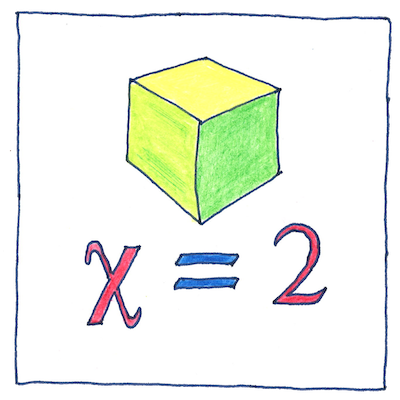# 1758 Euler characteristic

## The book of science

Tom Sharp

 Leonhard Euler mathematics

## Euler characteristic

• The Euler characteristic or χ
• (a Greek letter pronounced like “sky”
• but without the “s”)
• of any geometric object
• is the number of vertices
• (corners)
• minus the number of edges
• plus the number of faces.
• The χ of any convex polyhedron
• (any three-dimensional geometric object
• with sharp vertices, straight edges, and flat faces)
• is 2.
• The χ of a cube is 2
• (8 vertices - 12 edges + 6 faces).
• The χ of a triangular pyramid is also 2
• (4 vertices - 6 edges + 4 faces).
• But the χ of nonconvex polyhedra
• is more interesting.
• The χ of a tetrahemihexahedron is 1
• (6 vertices - 12 edges + 7 faces).

## Topological invariant

• Let me tell you about the χ of a sphere.
• You might think that the χ of a sphere would be 1
• (0 vertices - 0 edges + 1 face);
• however, the χ of a sphere is 2.
• To understand this, first we need
• to realize no matter how you cut it,
• a sphere always remains a sphere.
• So cut it both horizontally and vertically.
• The two cuts cross each other in two places;
• these are its two vertices.
• The cuts also divide it into 4 faces that share 4 edges.
• Its χ is therefore (2 - 4 + 4) = 2.
• Cut the sphere vertically again
• at a right angle to the previous vertical cut.
• Now the sphere has 6 vertices, 12 edges, and 8 faces,
• retaining its χ of 2.
• No matter how you cut it,
• a sphere will always have a χ of 2.
• You can cut and flatten faces into any convex polyhedron
• and it still keeps its χ of 2.